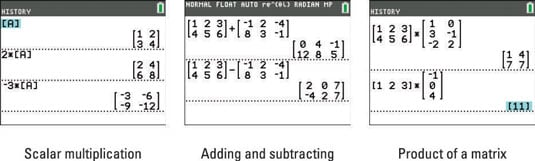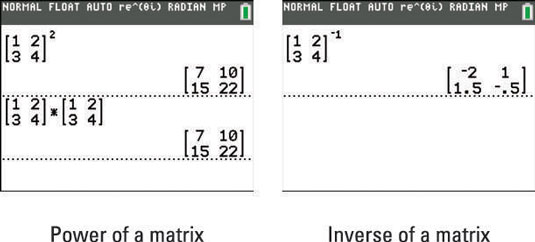##### TI-84 Plus CE Graphing Calculator For Dummies, 3rd EditionYou can use your TI-84 Plus calculator to perform matrix arithmetic. When evaluating arithmetic expressions that involve matrices, you usually want to perform the following basic operations: scalar multiplication, addition, subtraction, and multiplication. You might also want to raise a matrix to an integral power.

Be careful! Matrix arithmetic is not like the arithmetic you’ve been doing for years. Expect the unexpected! Multiplying two matrices is quite different than multiplying two numbers.

Here’s how you enter matrix operations in an arithmetic expression:

1. Enter a matrix on the Home screen.

To paste the name of a matrix into an expression, press [2nd][x–1] and key in the number of the matrix name. Alternatively, you can press [ALPHA][ZOOM] to quickly create a new matrix.

2. Enter the operations you want to perform and press [ENTER] when you’re finished.

Here’s how you enter the various operations into the arithmetic expression:

• Entering the scalar multiple of a matrix: To enter the scalar multiple of a matrix in an arithmetic expression, enter the value of the scalar and then enter the name of the matrix, as shown in the first screen.• Adding or subtracting matrices: When adding or subtracting matrices, both matrices must have the same dimensions. If they don’t, you get the ERROR: DIMENSION MISMATCH error message.

Entering the addition and subtraction of matrices is straightforward; just combine the matrices by pressing + or –, as appropriate. The second screen illustrates this process.

• Multiplying two matrices: When finding the product A*B of two matrices, the number of columns in the first matrix (matrix A) must equal the number or rows in the second matrix (matrix B). If this condition isn’t satisfied, you get the ERROR: DIMENSION MISMATCH error message.

Matrix multiplication is a tricky process. However, entering matrix multiplication in a calculator is straightforward; just multiply the matrices by pressing [x], as shown in the third screen.

• Raising a matrix to a positive integral power: When finding the power of a matrix, the matrix must be square (number of rows = number of columns). If it isn’t, you get the ERROR: INVALID DIMENSION error message.

Only non-negative integers can be used for the power of a matrix. If the exponent is a negative integer, you get the ERROR: DOMAIN error message.

Look at the top of the first screen. Is that the answer you expect to get when you square a matrix? It’s better to think of squaring a matrix as multiplying a matrix by itself, as shown at the bottom of the first screen.• Finding the inverse of a matrix: When finding the inverse of a matrix, the matrix must be square (number of rows = number of columns) and nonsingular (nonzero determinant). If it isn’t square, you get the ERROR: INVALID DIMENSION error message. If it is singular (determinant = 0), you get the ERROR: SINGULAR MATRIX error message.

Enter the inverse of a matrix by entering the matrix and then pressing [x–1], as shown in the second screen.

It may look like you’re putting a matrix to the power of –1 when your press [x–1]. That isn't the case! In this setting, [A]–1 is read as “the inverse of matrix A” or “inverting matrix [A].” This is similar to the notation that’s used for inverse functions.